# Scheduling Job Shops with Batch Machines Using the Lagrangian Relaxation Technique

Download 420.03 Kb.
 Page 1/3 Date 09.06.2018 Size 420.03 Kb.
1   2   3
 For submission to the European Journal of Control Scheduling Job Shops with Batch Machines Using the Lagrangian Relaxation Technique Jihua Wang and Peter B. Luh The University of Connecticut Dept. of Electrical & Systems Engineering Storrs, CT 06269-2157, USA Phone: (860) 486-4821 Fax: (860) 486-2447 Email: Jihua@brc.uconn.edu, Luh@brc.uconn.edu Abstract Scheduling is a key factor for manufacturing productivity. Effective scheduling can improve on-time delivery, reduce work-in-process inventory, cut lead time, and improve machine utilization. Motivated by the extensive use of batch machines in manufacturing industries, the scheduling of job shops with batch machines is studied in this paper. Unlike machines that can process one part at a time (called "standard machines" for simplicity), a batch machine is the one that can simultaneously process several parts with the same processing requirement as a batch, subject to the capacity of the batch machine. This simultaneous processing requires the "synchronization" (batch formation) of different parts for a batch operation. In view that a part may have to be processed by many standard and batch machines, multiple batch formation and batch splitting result in complicated coupling among the parts. In this paper, batches are viewed as "virtual" facilities that host and "synchronize" parts for processing, and compete for batch machine capacity. A novel integer programming formulation with a "separable" structure is then obtained. To solve this problem, a Lagrangian relaxation approach is used to decompose the problem into part and batch subproblems that can be solved by using an efficient dynamic programming algorithm. The multipliers are updated at the high level by using an “interleaved conjugate gradient method,” and a heuristic is developed to obtain feasible schedules based on subproblem solutions. Numerical testing shows that the integrated consideration of batch formation and sequencing results in high quality schedules, and the algorithm is computationally efficient to solve practical problems. Keywords: Scheduling, Job shop, Batch machine, Lagrangian relaxation 1. INTRODUCTION Scheduling is a key factor for manufacturing productivity. Effective scheduling can improve on-time delivery of products, reduce work-in-process inventory, cut lead times, and improve machine utilization. In discrete manufacturing systems, a machine often processes only one part at a time (called "standard machine" for simplicity). There are also many "batch machines," where a batch machine is the one that can simultaneously process multiple parts with the same processing requirement as a batch, subject to the capacity of the batch machine. For example, a heat-treat oven can simultaneously process multiple parts with the same processing requirement (temperature, processing time etc.) in a batch. A 3-axle NC machine can perform the same operation of up to three parts simultaneously. The high utilization requirement and the long non-preemptive processing times place great efficiency demand on these machines. Motivated by the extensive use of batch machines in discrete manufacturing systems and the associated demand on high efficiency, this paper presents an optimization-based method for the scheduling of job shops with both standard and batch machines. The scheduling of batch machines requires the grouping of parts into batches (batch formation) and the sequencing of batches. Parts with the same processing requirement belong to a "group," and parts from different groups cannot be processed in the same batch. Since a batch machine can simultaneously process multiple parts, it may be desirable to form a batch with as many parts as possible. However, parts assigned to a batch may not be available at the same time, and the parts available earlier have to wait for processing until all the parts become available. This waiting may cause significant delay of the parts available earlier, resulting in poor scheduling performance. The trade-off between utilization and due date performance has been observed to be a major difficulty for many factories (Zijm, 1995). To overcome the difficulty, it is required to consider batch formation and sequencing in an integrated fashion. However, in view that a part may have to be processed by many standard and batch machines, multiple batch formation and batch splitting result in complicated coupling among these parts. Literature Review In view of the difficulties in scheduling batch machines, the problem is often separated into batch formation and batch sequencing problems. In Ahmadi et al. (1992), the scheduling of a two- or three-machine flow shop with one batch machine is considered as a two-stage batch formation and sequencing problem. Makespan and the sum of job completion times are used as the performance criteria, and a heuristic method is presented. A two-stage batch formation and batch sequencing algorithm for NC punch presses is developed in Lee et al. (1993). At the first stage, batches are formed to maximize the number of parts in batches and the tool magazine utilization. At the second stage, batches are sequenced to reduce the total setup time by using the "nearest neighbor" heuristic. Methods for the integrated consideration of batch formation and sequencing have also been presented. The scheduling of parts belonging to multiple groups on a batch machine are considered in Uzsoy (1995). The objectives of makespan, maximum lateness and total weighted completion time are discussed. It is shown that when all parts are available simultaneously, keeping a batch as full as possible gives the optimal solution. Several heuristics are developed to minimize the maximum lateness for dynamic job arrivals. In Dessouky et al. (1996), a mixed integer nonlinear model with the objective to minimize the total tardiness is developed for the scheduling of batch chemical plants. A heuristic algorithm is developed to determine the sizes of batches and their schedule to satisfy the demand of outstanding orders. Optimization-based approaches have also been reported. In Liao et al. (1993), a combined Lagrangian relaxation and linear network flow algorithm is developed for the scheduling of a flow line of semiconductor wafer fabrication with batch machines. Batch formation is to determine the batch size, subject to the capacity of the batch machine and flow balance equations. In Wang et al. (1994), the scheduling of a single batch machine is studied. A separable problem formulation accommodating both batch formation and batch sequencing is presented. The large number of constraints involved, however, limits its application to problems of small sizes. Overview of the Paper In this paper, the scheduling of a job shop with batch machines is studied with an integrated consideration of batch formation and sequencing. Because of the combinatorial nature of the problem, it is very difficult to obtain optimal schedules, especially within a limited amount of computation time. The goal of the study, therefore, is to obtain near-optimal schedules with quantifiable quality in a computationally efficient manner. To achieve this, a Lagrangian relaxation based method is developed to decompose the problem into smaller subproblems that can be efficiently solved without encountering complexity difficulty. The key for the effective application of Lagrangian relaxation is to have a problem formulation with a “separable” structure  the objective function and all "coupling constraints" are additive in terms of basic decision variables. The synchronization, however, leads to the strong couplings among parts, making it difficult to have a separable formulation. To overcome the difficulties, batches are viewed as "virtual" facilities that host and "synchronize" parts for processing, and compete for batch machine capacity. The hosting of parts and the competition for batch machines are delineated by two sets of linear constraints. A separable integer programming formulation with manageable numbers of variables and constraints is then obtained in Section 2. In Section 3, a solution methodology based on the synergistic combination of Lagrangian relaxation and heuristics is developed. Within the Lagrangian relaxation framework, dynamic programming (DP) is used to solve subproblems as in Chen, Chu and Proth (1995). The multipliers are updated at the high level by using the "interleaved conjugate gradient (ICG) method," and a heuristic is developed to generate feasible schedules based on subproblem solutions. The solution method has been implemented using the object-oriented programming language C++. Numerical testing presented in Section 4 shows that the integrated consideration of batch formation and sequencing results in high quality schedules, and the algorithm is computationally efficient to solve practical problems. 2. PROBLEM FORMULATION The formulation to be presented is built on our previous work on job shop scheduling (Hoitomt et al., 1993) and single batch machine scheduling (Wang et al., 1994) while considering multiple batch machines and the interaction among standard and batch machines. A general description of the problem is provided in Subsection 2.1. The constraints and objective function are then presented in Subsections 2.2, 2.3 and 2.4. A list of symbols used is provided in Appendix A for easy reference. 2.1 General Description According to processing capabilities, machines in the shop are grouped into a set of machine types, denoted by H, where a machine type h  H may consist of a few identical machines. The set for all batch machine types is HB, and the set for all standard machine types is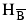. The number of machines available for a machine type h, denoted as Mhk, may change over a discretized time horizon K with time index k ranging from 0 through K - 1. For a batch machine of type h, the number of parts in a batch is restricted by the batch volume Vh of machine type h (e.g., the volume of a heat treat oven or the maximum number of parts on an NC machine). There are I parts to be scheduled HHH. Part i (i = 0, 1, …, I - 1) has a desired release time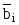and a given due date Di, and it consists of Ji non-preemptive operations. Operation j (j = 0, 1, …, Ji - 1) of part i, denoted as operation (i, j), requires a machine of type h belonging to a set of eligible machine types Hij to process for Pijh amount of time. Operations to be performed on standard machines are called "standard operations," and operations on batch machines are called "batch operations." All operations that can be processed on a batch machine type h  HB belong to Gh groups, and each operation occupies the batch volume Vh with a size (or a volume) vij. Operations belonging to group g (g = 0, 1, …, Gh - 1) are processed in Nhg batches, each requiring Phg amount of processing time, and Phg = Pijh if operation (i, j) belongs to the group. The batches indexed by n (n = 0, 1, …, Nhg - 1) are assigned to individual machines, and batches on each machine are processed in the ascending order of n. The number of batches within a group is given, and is assumed to be large enough to hold all batch operations of the group. Batch n (n = 0, 1, …, Nhg - 1) of group g on machine type h is denoted as batch (h, g, n), and group g on machine type h denoted by (h, g). In formulating the scheduling problem, batches are viewed as virtual facilities that host and synchronize operations on parts. The synchronization among operations is modeled as that between operations and the virtual facilities. To assign an operation to a batch machine type with beginning time k, a virtual facility (batch) should be available to host the operation, and the volume sum of the operations should not exceed the batch volume. Batches are also viewed as "virtual" operations that compete for batch machine capacity, and a batch machine can process at most one virtual operation at a time. Different from the above batch operations, operations performed on standard machine types directly compete machine capacity, that can be modeled as in Hoitomt, Luh, and Pattipati (1993). 2.2 Modeling of Standard Machine Types The machine capacity constraints for standard machines state that the number of operations assigned to a standard machine type h cannot exceed the number of type h machines available at time k, i.e.,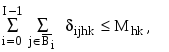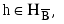k = 0, 1, ... , K - 1, (1) where ijhk is a 0-1 operation in-processing variable. It is 1 if operation (i, j) is performed on a machine of type h at time k, and 0 otherwise. Set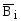is the set of all the standard operations of part i. Although the number of operation in-processing variables ijhk is huge, these variables are not independent decision variables. They are determined once the machine types for individual operations are selected, and operation beginning times are determined, i.e., ijhk =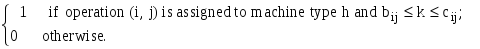(2) 2.3 Operation Precedence Constraints and Processing Time Requirements The operation precedence constraints state that an operation cannot start until its preceding operation is finished, i.e., cij + Sij -1  bi,j+1,  (i, j), (7) where cij is the completion time of operation (i, j), and bi,j+1 the beginning time of operation (i, j+1). The parameter Sij is the required "timeout" between operations (i, j) and (i, j+1), representing the processes not explicitly modeled in the problem formulation (e.g., the transportation time required in-between the two operations). The operation processing time requirements state that each operation must be assigned the required amount of processing time Pijh on the machine type selected, i.e., cij = bij + Pijh - 1, i = 0, 1, …, I - 1; j = 0, 1, …, Ji - 1 and h  Hij. (8) With processing times specified, operation completion times cij can be eliminated from the problem formulation. For notational convenience, they still appear in later derivation. 2.4 Modeling of Batch Machine Types As mentioned in Section 1, parts to be performed in a batch should be synchronized to have the same operation beginning and completion times. By viewing batches as virtual facilities, the difficult synchronization among these parts can be accomplished by synchronizing individual parts to batches. The batch constraints thus state that the total volume of parts beginning at time k for a batch operation may not exceed the total volume of batches beginning at time k, i.e.,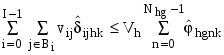, h  HB, g = 0, 1, ... , Gh - 1, k = 0, 1, ..., K-1, (3) where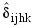is a 0-1 operation beginning variable. It equals 1 if operation (i, j) starts on machine type h at time k (i.e., bij = k), and 0 otherwise. Similarly,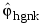is a 0-1 batch beginning variable. It equals 1 if batch (h, g, n) starts at time k (i.e., bhgn = k), and 0 otherwise. Since batches act as a virtual parts that compete batch machines, the machine capacity constraints for batch machines state that the number of batches performed on a batch machine type h may not exceed the number of batch machines available, i.e.,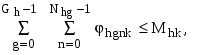h  HB, k = 0, 1, ... , K-1, (4) where hgnk is a 0-1 batch in-processing variable. It is 1 if batch (h, g, n) is performed on a machine of type h at time k, and 0 otherwise. Similar to the operation in-processing variables ijhk defined by (2), the operation beginning variables, the batch beginning variablesand the batch in-processing variables hgnk are not independent decision variables. They can be determined by the machine types for individual operations and operation beginning times. The batch precedence constraints provide the processing sequence of batches on each machine, i.e., chgn + 1  bhg,n+1, h  HB, g = 0, 1,..., Gh - 1, (5) where chgn is the completion time of batch (h, g, n), and bhg,n+1 the beginning time of batch (h,g,n+1). The batch processing time requirements state that each batch must be assigned the required amount of processing time Phg, chgn = bhgn + Phg - 1, h  HB, g = 0, 1, …, Gh - 1, n = 0, 1, …, Nhg - 1. (6) 2.5 Objective Various objective functions such as makespan have been used in literature. Study of practical scheduling, however, shows that the tardiness objective is likely to be more useful than other criteria such makespan (Blackstone et al., 1982). In addition, the additivity of the tardiness objective function facilitates the decomposition approach. The following objective function of weighted part tardiness and earliness is used to model the goal of on-time delivery and low work-in-process inventory. J 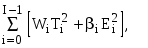(9) where Ti = max [0, ci,Ji-1-Di] is the tardiness, and Ei = max [0,- bi0] the earliness. The coefficient Wi is the tardiness weight of part i, and i the earliness weight. The overall problem is therefore to minimize the part tardiness and earliness penalty function (9), subject to the above machine capacity, batch, operation and batch precedence constraints. The decision variables are the machine types {hij} for individual operations, operation beginning times {bij}, and batch beginning times {bhgn}. Once these decision variables are determined, other variables {cij}, {ijhk}, {}, {ijhk}, and {} can be easily derived. Among the constraints, machine capacity and batch constraints are "coupling constraints" as they couple together decision variables associated with different parts and batches. Since all the coupling constraints and the objective function are additive in terms of decision variables, the problem formulation is separable. Lagrangian relaxation can therefore be effectively used to solve it, as will be presented in the next Section. SOLUTION METHODOLOGY Lagrangian relaxation (LR) is a mathematical programming technique for performing constrained optimization. It has been successfully applied to various manufacturing systems to obtained good schedules with quantifiable quality in a reasonable amount of computation time (Hoitomt, et al., 1993; Liao et al., 1993; Luh et al., 1995). Recently, the embedding of dynamic programming within the Lagrangian relaxation framework for solving subproblems has improved algorithm convergence and schedule quality (Chen et al., 1995; Kaskavelis et al., 1995 and Wang et al., 1997). Similar to the pricing concept of a market economy, the Lagrangian relaxation method replaces "hard" coupling constraints (e.g., the machine capacity constraints) by the payment of a certain "prices" (i.e., Lagrange multipliers) for the use of machines at individual time units. The original problem can thus be decomposed into many smaller subproblems. These subproblems are much easier to solve as compared to the original problem, and solutions can be efficiently obtained by using "dynamic programming." These prices or multipliers are iteratively adjusted based on the degree of constraint violations following again the market economy concept (i.e., increase the prices for over-utilized time units and reduce the prices for under-utilized time units). Subproblems are then re-solved based on the new set of multipliers. In mathematical terms, a "dual function" is maximized in this multiplier updating process, and the dual costs (values of the dual function) are lower bounds to the optimal feasible cost. At the termination of such price updating iterations, a few constraints may still be violated when subproblem solutions are put together. Simple heuristics are thus applied to adjust subproblem solutions to form a feasible schedule satisfying all constraints. Heuristics can also be run after selected optimization iterations to check convergence status or to generate more candidate feasible schedules. Optimization and heuristics thus operate in a synergistic fashion to generate effective schedules. The quality of the feasible schedule can be quantitatively evaluated by comparing its cost to the largest lower bound provided by the dual function. 3.1 The Lagrangian Relaxation Framework Standard machine capacity, batch machine capacity, and batch constraints are first “relaxed” by using nonnegative Lagrange multipliers {hk, h }, {hk, h  HB} and {hgk}, respectively. The Lagrangian is formed as: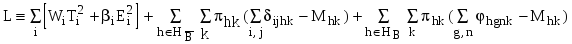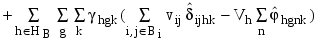. (10) In the above, {hk, h } are the prices for performing operations on standard machines, and {hk, h  HB} the prices for processing batches. The multipliers {hgk} are the prices for an operation to occupy the batch volume. With the multipliers given, the "relaxed problem" is to choose the decision variables to minimize the Lagrangian L subject to operation and batch precedence constraints. After regrouping relevant terms within L, the problem is decomposed into part and batch subproblems. 3.2 Part Subproblems and Their Resolutions Download 420.03 Kb.Share with your friends:
1   2   3

The database is protected by copyright ©ininet.org 2020
send message

Main page